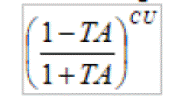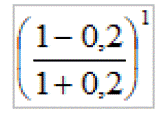# What Is The Risk Of Ruin In Trading (Probability Of Ruin And Loss)

Last Updated on July 7, 2022 by Oddmund Groette

The risk of ruin in trading is mostly ignored by traders. Most traders are optimists, but the difference between defense and attack is often tiny. What is the risk of ruin in trading? Can the risk of ruin in trading be calculated?

The risk of ruin in trading can be calculated by using certain formulas that require some assumptions. The formulas are not perfect, but they are approximations.

The formulas provided in this article are theoretical and must be treated with caution. No formula can ever capture an uncertain future! There are many advantages with backtesting but the tests might be based on luck or being curve fitted. Besides, black swans are per definition (negative) surprises. However, backtests are useful for approximation and understanding.

Why is it important to understand the risk of ruin?

Because ruin means financial loss. The balance between initial capital, the extent of your trading edge, and the variability of the underlying price, has been the foundation of any speculative endeavors for thousands of years. How much should you bet on each trade without facing too much of a risk of ruin?

## What is the most important in trading?

We have repeatedly said that the most important thing in trading is having a trading edge. Psychology is overrated in trading  – you need a statistical edge before you can think about working on your trading biases and risk management.

These are the most important things to understand in trading:

1. Having a trading edge.
2. Know yourself and your limit. You need a trader’s mindset.
3. You need proper risk management.

Risk of ruin is all about risk management – number three on our list. Both 2 and 3 are less important than number one.

Why?

Because numbers 2 and 3 will never be able to replace a negative trading edge. Thus, you need solid, robust, and profitable trading edges before you can think about numbers 2 and 3!

That out of the way, let’s return to the subject of the article:

## What is the risk of ruin in trading?

Risk of ruin is the probability or likelihood of losing all your capital or being limited from further trading. We define risk of ruin as the chances or probability that you will suffer losses that force you to stop or be unable to recover. It doesn’t necessarily mean that you lose everything.

On the contrary, evidence points to traders losing faith even at the smallest drawdowns. Very few traders are able to go through a drawdown in trading without giving up. When the drawdown ticks above 20% most traders start curve fitting their models or abandoning them. Everything looks easy in a backtest when you know the end result! Backtesting works, but only to a certain degree.

Risk of ruin is a concept that is, by now, well understood in most forms of gambling. If you play cards or roll dice, you can calculate risk of ruin accurately (assuming no foul play).

Let’s make a simple example:

Let’s assume you have 500 dollars and want to bet 100 per game where you have a 50% chance of winning or losing an equal amount. This means you are at risk of ruin with five games or more. Theoretically, you can lose five times in a row from the start, and thus be flat broke. The probability for that is 3.125%. That might sound small, but you are unable to continue playing more if you lose all your money.

But in finance, risk of ruin is not as easy to determine as in the example above. Calculation of risk is complex and has many unknown factors.

## Why do you want to avoid risk of ruin?

If you lose 50% of your assets you need to make 100% to recover. If you lose 75% you need to make 400%. The math makes it pretty easy to see why you want to avoid any substantial losses.

And, as mentioned above, even with smaller losses and drawdowns most traders simply give up.

## How to calculate the risk of ruin

There are many ways to calculate the risk of ruin – depending on the complexity. However, we get a long way by using even the simplest methods. The main purpose of this article is to show how liable you are to adverse movements on your bankroll.

#### Gambler’s ruin

Let’s assume you have a very simple trading strategy:

1. The win ratio is 60% (and thus the loss ratio is 40%).
2. You have an equal chance of winning or losing the same amount.

Let’s assume you have 50 000 and want to bet this amount on each trade.

What is the chance of going broke if you allocate 50 000 of your equity for each trade?

After one trade the probability of ruin is obviously 40%.

What if you make a gain on the first trade? Then you have 100 000. This means you can lose on the two next trades:

0.6 * 0.4 * 0.4 = 0.096

There is a 9.6% chance of going bust if the first trade was a winner.

Then we can combine the probabilities to calculate risk of ruin after three trades. We find this by adding the risk of ruin on the first trade plus risk of ruin after three trades: 40% + 9.6% = 49.6%.

We can then calculate the risk of ruin after five trades:

• Ruin on the first trade
• Ruin on the third trade
• Ruin on the fifth trade

You can go broke on the fifth trade via two paths:

• Win, win, loss, loss, loss (0.6*0.6*0.4*0.4*0.4=0.023), or
• Win, loss, win, loss, loss (0.6*0.4*0.6*0.4*0.4=0.023)

Risk of ruin is hence 54.2% after five trades (40+9.6+2.3+2.3).

By doing this we can calculate the risk of ruin after N trades. This is, of course, a bit cumbersome. To help us out Wolf von Rönik provided a formula in Technical Analysis of Stocks and Commodities in July 2001 (the formula was also explained in Perry Kaufman’s Smarter Trading):TA = Percentage win ratio minus percentage loss ratio

CU = Your capital divided by the number of stocks and/or strategies

If we put our numbers into the formula it looks like this:This means you have a 67% probability of ruin!

What if you allocate just 50% of your capital? Risk of ruin drops to 44%. The more strategies you add, the less likely you are to go broke.

Here is the graph showing hows it pays off to diversify:The pink line shows the risk when the win ratio drops to 55%.

Let’s take the exercise one step further: if you have a stop-loss threshold at for example 30 000, how many trades can you make until you hit that level? How much should you risk per trade? That means you can lose 30 000 before you quit. We need to calculate the U in the formula above to define the risk per trade.

Let’s say you evaluate two options: one where you risk 5 000 per trade, and one where you risk 1 000 per trade. What is the probability of ruin? The U in the formula above is thus 6 (30 000/5 000) and 30 (30 000/1 000).

The risk of ruin is 13% if you risk 5 000 per trade but reduced to a negligible 0.0005% if you risk 1 000 per trade. This illustrates pretty well the trade-off between attack and defense in trading!

Obviously, the formula above is a bit far-fetched from reality because it doesn’t consider the amount you lose or gain per trade. Also, it only works if the win ratio is above 50%.

Let’s look at another method that is slightly closer to real-life trading:

#### An improved version of how to calculate risk of ruin

Ralph Vince made an improvement to the above formula in his book called Portfolio Management Formulas. It’s an old book, published in 1990, but the formula is still valid, of course. Vince proposes a bit more complex formula but it’s a bit more realistic.

Vince’s formula requires the following data and variables:

• The initial capital (100 000)
• Risk capital in percentage
• Win ratio and loss ratio
• The average winner per trade and the average loser per trade

The formula is like this:You can find your numbers from your trading strategy and put them into this formula to get an approximate estimation of how likely you are to go broke or get ruined.

## Monte Carlo simulations help you simulate risk of ruin

Luckily for us, there exists a better and much faster way of determining the risk of ruin: Monte Carlo simulations. Monte Carlo simulations are a statistical tool that measures the uncertainty of your trading strategy and look at different sequence risk. The logic is to look at different outcomes of the trades if they had taken a different order. Sequence risk is very important and something we have covered in two articles:

Monte Carlo simulations are a great way to understand the risk and uncertainty in your trading strategy. We recommend Mark Spitznagel’s Safe Haven Investing if you want to dig deeper into the subject.

## Why should you know risk of ruin?

Your capital is the most precious asset you have. If you lose it, you can’t make a comeback. A statistical edge is the most important thing in trading, but even a statistical edge can be useless if you don’t understand risk management.

Trading is all about understanding the three main elements we mentioned at the beginning of the article: having a trading edge, knowing yourself and your limits, and having proper risk management. All three elements are somewhat dependent on each other.

Even better, if you truly understand the risk you are undertaking, you improve your confidence in your strategies. You are less likely to abandon a strategy during a normal drawdown.

## How can you reduce your risk of ruin?

There are several things you can do to reduce the risk of ruin:

#### Know how much risk you take

The first thing you can do is to employ some simple metrics described in this article: throw in some numbers into the formulas or run some Monte Carlo simulations.

#### Trade smaller than you’d like

This is something we have advised for years. This has many benefits – not only reducing your risk of ruin – you most likely also minimize your most common trading biases.

#### Increase the win ratio

The win ratio in trading is important to improve your odds for success.

Very few traders can continue trading after x number of losses in a row, and thus a high win ratio is important.

#### Remove some trading strategies?

Do all your trading strategies add value to your portfolio of strategies? Start by making them all in one backtest and remove one by one from the overall result and see what happens to the overall result.

Too many traders use strategies that have overlapping trading and don’t give any real correlation benefits. Adding and removing strategies force you to look deeper into the matter.

Furthermore, you might increase both your win ratio and expected profits by reducing the number of trading strategies.

## Risk of ruin in trading – conclusions:

What do we learn from this simple exercise? The main takeaway is that diversification and margin of safety pay off if you want to avoid risk of ruin in trading.

Diversification into different time frames, assets, and market directions is the only holy grain in trading.

And, perhaps above all else, always err on the safe side. Trade smaller than you’d like and always have a margin of safety.